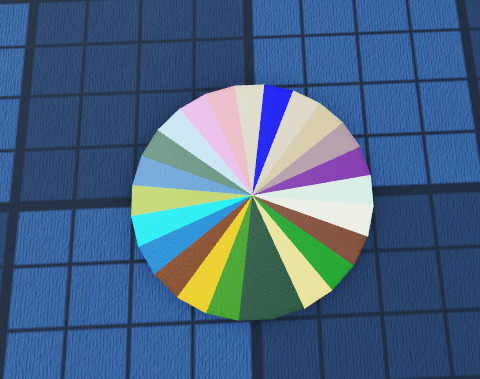# Generate Triangular Circle w/ Function

Sorry, could you explain what this is calculating exactly? I’m not sure why, but I have trouble understanding math when presented in a traditional way rather than a written algorithm in code.

Sure thing. `s` is the desired length of each equilateral side. `s/2` is the length of the short side of the right triangles. `h` is the length of the longer side of the right triangle.
To find `h`, you need to use the pythagorean theorem.

``````local side = 1 -- 1 stud equilateral sides
local x_axis = side/2 -- x direction for the Wedge.
-- Pythagorean theorem to find the last side of the wedge.
local y_axis = math.sqrt(x_axis^2 + side^2)
``````

Oh that’s right, I forgot the lengths aren’t exactly equal. Though I’m still not exactly sure what to do with this for this particular case. Sorry if I’m missing something obvious right now.

When looking at this circle, you’ll see that it’s not perfectly smooth (obviously). You can see the pointy bits, which are the corners of each triangle. I’m trying to figure out how to generate a circle this exact same way, but with individual parts for each triangle.

Not the easiest thing to see, but it seems that this particular circle is made up of 24 triangles, 6 per quarter circle. The diameter of this circle is 4, and therefor the radius is 2 (making the length of each triangle 2 studs, I assume). What this leaves me with, I think, is needing to calculate the width of each triangle. Oh and the rotation of each, but that’s probably just division.

I guess the width would be the circumference (which I think is `2*pi*radius`). I had to round up to the second decimal place as any lower would result in a gap. The rotation is just `360/numberOfTriangles`.

So this is what I wanted to accomplish:

I cheated by using Roblox’s cylinder to see how they did it. I counted it out to see that it had 24 triangles (6 in each quarter). I determined that the length of each triangle would be the radius, which was 2 studs in this case. To determine the width of each triangle, I did `2*pi*radius`, with the second decimal place rounded up to fill the gap. The rotation for each triangle was `360/numberOfTriangles` (24), which ended up being 15 degrees of rotation each.

The last thing I need to figure out is how to determine all of these values based on however many triangles I’m wanting to use, which I’m still trying to figure out. I want to write a function that can generate a circle via triangles with however many triangles I want.

I am typing something up that might work.
like this?``````local thickness = .2 --how thick the circle is
local slices = 24
local pos = Vector3.new(5,0,0) --where to draw the circle

local output = workspace:FindFirstChild("Output")
if not output then
output = Instance.new("Folder")
output.Name = "Output"
output.Parent = workspace
end
output:ClearAllChildren()

local deg = 360 / slices

local wedge = Instance.new("Part")
wedge.Anchored = true
wedge.CanCollide = false
local specialMesh = Instance.new("SpecialMesh")
specialMesh.MeshType = Enum.MeshType.Wedge
specialMesh.Parent = wedge

for n = 1,slices do
local w = wedge:Clone()
w.BrickColor = BrickColor.random()
w.PivotOffset = CFrame.new(0,- (a/2) ,- (radius/2))
w.Parent = output
w = w:Clone()
w.Parent = output
end

``````

This site is what helped me figure it out

1 Like

That does appear to be what I’m going for. I will mark this as a solution as it’s essentially what I was originally going for. Although some shower thoughts have made me realize that resizing triangles based on the raycast distance wouldn’t have the best looking outcome. I realized that instead, I could just take the points from the raycasts, then create triangles between all of them. The end result should be a visualized radius of what can be reached and what can’t. Once I get that working, I’ll edit this with a screenshot of it (if I remember to).

1 Like

how do you do it?
this is very interesting stuff

I essentially just shot a bunch of raycasts out and drew triangles between 2 hit points and the center. The red triangles were similar, but for the maximum distance of the ray to the hit points (where the white ends early).

This topic was automatically closed 14 days after the last reply. New replies are no longer allowed.